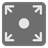Expand Intervals
Simple
English

An interval of consecutive positive integers can be succinctly described as a tuple that contains first and last values. For example, the interval that contains the numbers 5, 6, 7, 8, 9 can be described as (5,9). Multiple intervals can be united together into iterable. Given an an iterable with intervals (guaranteed not to overlap with each other and to be listed in a sorted ascending order), create and return the list that contains all the integers contained by these intervals.

Input: The iterable of tuples of two integers.

Output: The iterable of integers.

Example:

```expand_intervals([(1, 3), (5, 7)]) == [1, 2, 3, 5, 6, 7]
expand_intervals([(1, 3)]) == [1, 2, 3]
```

Precondition: Each element in the interval is an integer and

The mission was taken from Python CCPS 109 Fall 2018. It’s being taught for Ryerson Chang School of Continuing Education by Ilkka Kokkarinen